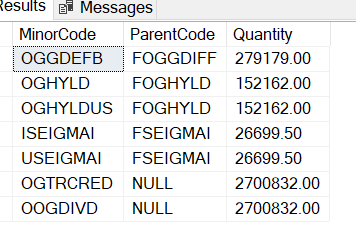# SUM function to SUM based on a condition

Hi have a very simple table with data. I need to extract the Quantities for each MinorCode but these quantities should be the sum of the quantities only if a MinorCode has the same ParentCode and not the Quantities as per each record in the table.
For example MinorCodes OGHYLD and OGHYLDUS share the same ParentCode FOGHYLD therefore in my results I need to see the sum total of 152,162 (81,260 plus 70,902) as the Quantity for OGHYLD and OGHYLDUS. Some records to dot share a ParentCode therefore no SUM required.

Table data & Expected results:

I have tried a simple sum and group by but it does not give me the correct results.

SELECT MinorCode as Code, sum(Quantity) AS Quantity
FROM tbl.data
GROUP BY Parent

How would I specify the above requirement in order to show the SUM of the respective MinorCode Quantities if they share the same ParentCode?

If you post directly usable data -- CREATE TABLE and INSERT statement(s) to load the data in the picture -- then I/we can write a query against that data.

Hi ScottPletcher

``````create table #data
(
MinorCode VARCHAR(10)
,	ParentCode VARCHAR(10)
,	Quantity DECIMAL(20,2)
)

insert into #data
values
('OGHYLD','FOGHYLD','81260'),
('OGHYLDUS','FOGHYLD','70902'),
('ISEIGMAI','FSEIGMAI','12145.5'),
('USEIGMAI','FSEIGMAI','14554'),
('OGGDEFB','FOGGDIFF','279179'),
('OGTRCRED','NULL','268507'),
('OOGDIVD','NULL','2432325')

select * from #data
``````

The aim is to sum the Quantities for each MinorCode where the respective ParentCodes are the same. E.g. for OGHYLD and OGHYLDUS the Quantity for both of them should be the sum of 81,260 + 70,902 thus 152,162 as both of them have the same ParentCode of FOGHYLD.

Expected output:

hope this helps ..

``````; with cte as
(
SELECT
ParentCode
,  sum(Quantity) AS Quantity
FROM
data
GROUP BY
ParentCode
)
select
a.MinorCode
, a.ParentCode
, b.Quantity
from
data a
join
cte b
on a.ParentCode = b.ParentCode
``````Thank you harrishgg1

It works perfectly except where the MinorCodes that do not have a ParentCode (NULL). The group by group these together as well which is wrong. I tried to do a case statement in the group by but it's not working.

``````group by case
when ParentCode is not null then ParentCode
end
``````

hopefully this

`````` ; with cte as
(
SELECT
isnull(ParentCode,1111111) as ParentCode
,  sum(Quantity) AS Quantity
FROM
data
GROUP BY
isnull(ParentCode,1111111)
)
select
a.MinorCode
, isnull(a.ParentCode,1111111) as ParentCode
, b.Quantity
from
data a
join
cte b
on a.ParentCode = b.ParentCode``````
``````;WITH CteParentTotals AS (
SELECT ParentCode, SUM(Quantity) AS ParentQuantity
FROM #data
WHERE ParentCode <> 'NULL' AND ParentCode IS NOT NULL
GROUP BY ParentCode
)
SELECT d.MinorCode, d.ParentCode, ISNULL(CPT.ParentQuantity, d.Quantity) AS Quantity
FROM #data d
LEFT OUTER JOIN CteParentTotals CPT ON CPT.ParentCode = d.ParentCode``````

Whatever you do, don't use a numeric constant against a varchar column! That will force the column to be converted to a number and, if any column can't be converted to a number, will cause the query to fail.

I hate the idea of adding a "magic" ParentCode, but if you do decide to, at least use quotes around it:

isnull(ParentCode,'1111111')

thank you for

pointing it out Scott